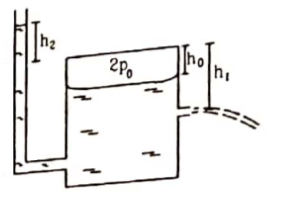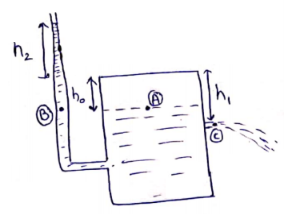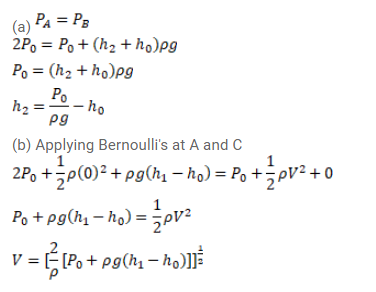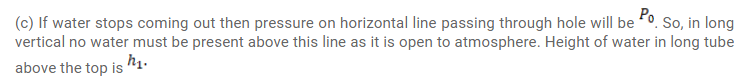Deepak Scored 45->99%ile with Bounce Back Crack Course. You can do it too!

# Use R=8.3 J/mol-K wherever required. Figure shows a large closed cylindrical tank containing water.

Question:

Use $\mathrm{R}=8.3 \mathrm{~J} / \mathrm{mol}-\mathrm{K}$ wherever required.

Figure shows a large closed cylindrical tank containing water. Initially the air trapped above the water surface has a height ${ }^{h_{0}}$ and pressure ${ }_{2} p_{0}$ where $p_{0}$ is the atmospheric pressure. There is a hole in the wall of the tank at a depth $h_{1}$ below the top from which water comes out. A long vertical tube is connected as shown.

(a) Find the height $h_{2}$ of the water in the long tube above the top initially.

(b) Find the speed with which water comes out of the hole.

(c) Find the height of the water in the long tube above the top when the water stops coming out of the hole.Solution: